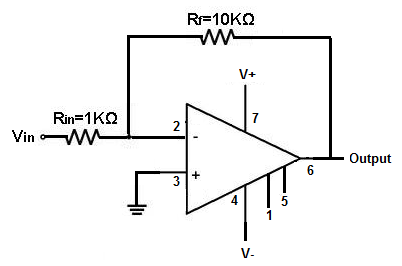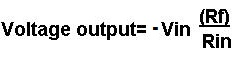﻿ How to Connect the LM741 Op Amp Chip to a Circuit# How to Connect the LM741 Op Amp Chip to a Circuit

In this article, we'll explain how to connect a LM741 Op Amp Chip to a circuit to allow for amplification.

A LM741 Op Amp is a versatile chip that can be made to do a variety of tasks such as a variety of math functions as well as amplification.

In this lesson, we will use it as an amplifier.

### Pinout

A LM741 is a 8-pin DIP chip, meaning it has 8 pins all having their different functions.

Below is the pinout diagram of a LM741 Op amp chip:Pin 1: Offset Null- This pin will be left open
Pin 2: Inverting Input- This is where the positive part of the input signal that we want to amplify goes if we want our amplified signal inverted. If we don't want it inverted, we place the positve part of the signal into the Non-inverting terminal and place the negative or ground part of our signal here.
Pin 3: Non-inverting Input- This is where the positive part of the input signal that we want amplified goes if we want our signal non-inverted. Pin 4: V-- The LM741 Op amp is a dual power supply op amp, meaning it must be supplied positive DC voltage and negative DC voltage. Pin 4 is where the op amp gets supplied with negative DC voltage.
Pin 5: Offset Null- This pin will be left open.
Pin 6: Output- This is the terminal where the output, the amplified signal, comes out of. Whatever output the amplifier will drive gets connected to this terminal.
Pin 7: V+- This is the terminal which receives the positive DC voltage.
Pin 8: NC- This pin will be left open.

We will now wire up the LM741 so that it provides amplification by a factor of 10.

This is how the circuit of the LM741 Op Amp will be connected in a circuit to provide an amplification of 10, meaning the output signal will be 10 times larger than the input signal.Place +15VDC to pin 7 and -15VDC to pin 4. This is necessary so the op amp can have bias power to allow it to perform amplification. Without this DC power, the op amp cannot work.

When you connect the signal input to the inverting side of an op amp, the voltage output is equal to:The gain of the op amp is equal to:If you want to increase the gain of the op amp, all you have to do is put a larger resistance for Rf. If you want to decrease the gain of the op amp, you just put a lower resistance value for Rf.

This is how you connect a LM741 Op amp for amplification.

You can use our online Op Amp Gain Calculator to calculate how much gain you want the op amp LM741 op amp circuit to produce.

Related Resources